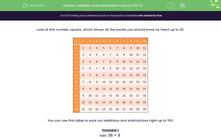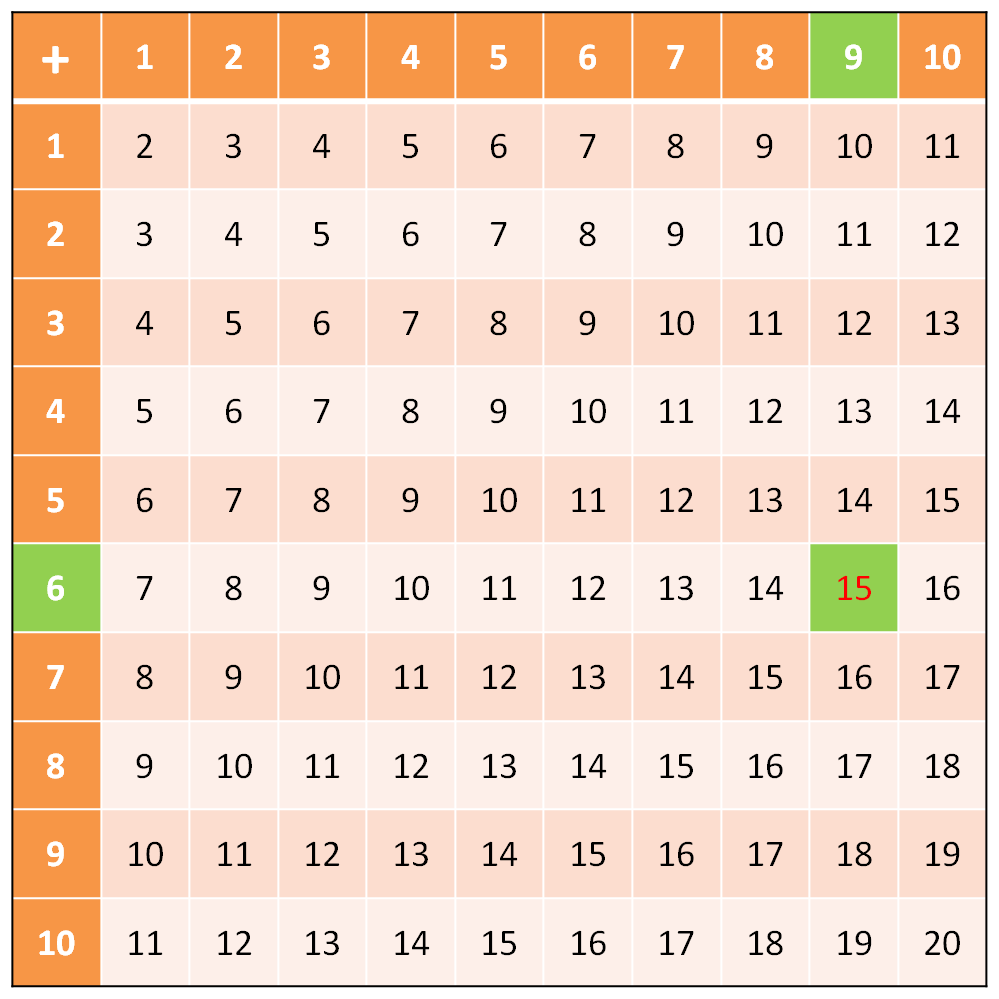# Know Addition and Subtraction Facts to 100

In this worksheet, students will practise their addition and subtraction number bonds to 20 and use them to derive related facts to 100.Key stage:  KS 1

Curriculum topic:   Number: Addition and Subtraction

Curriculum subtopic:   Use Addition/Subtraction Facts to 100

Popular topics:   Subtraction worksheets, Addition worksheets

Difficulty level:#### Worksheet Overview

Look at this number square, which shows all the bonds you should know by heart up to 20.You can use this table to help to work out additions and subtractions right up to 100.

Example 1

Method 1:

Split 36 into 3 tens and 6 ones.

Add the ones together: 6 + 9 = 15   This is where the number square above would help you!Now add 30 + 15 together. You could split 15 into 1 ten and 5 ones: 30 + 10 = 40   and then 40 + 5 = 45

Method 2:

Can you make the calculation easier by slightly changing it?

In this example, you are adding 9. How much easier would it be if you added 10?

Let's try: 36 + 10 = 46. Easy right?

But, we were supposed to add only 9! So, simply subtract 1 and you've got your answer: 46 - 1 = 45

Example 2

Do this subtraction:  15 - 9

Method 1:

Look at the number square. When you subtract, the starting number is inside the square.

Find 15 in the square.

Follow the lines and columns to the numbers that add together to make 15, and you get 6 and 9.

So, 15 - 9 = 6Method 2:

Can you make the calculation easier by slightly changing it?

In this example, you are subtracting 9. How much easier would it be if you subtracted 10?

Let's try: 15 - 10 = 5

But, we were supposed to subtract 9! So, simply add 1 and you've got your answer: 5 + 1 = 6

So, 15 - 9 = 6

Can you see that there is often more than one way of working out the answer to addition and subtraction problems? Just find the way that works best for each question.Let's have a go at some questions now.

If you want to look at the 100 square while you're doing the questions, just click on the red help button on the screen.

### What is EdPlace?

We're your National Curriculum aligned online education content provider helping each child succeed in English, maths and science from year 1 to GCSE. With an EdPlace account you’ll be able to track and measure progress, helping each child achieve their best. We build confidence and attainment by personalising each child’s learning at a level that suits them.

Get started